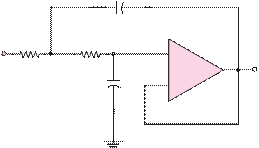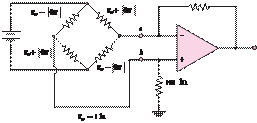### Create an Account

Already have account?

### Forgot Your Password ?

Home / Questions / Find an expression for the gain of the circuit of Figure P823 821 Differential a...

# Find an expression for the gain of the circuit of Figure P823 821 Differential amplifiers are often used in conjunction with the Wheatstone bridge Consider the bridge shown in Figure

Find an expression for the gain of the circuit of Figure P8.23.

8.21  Differential amplifiers are often used in conjunction with the Wheatstone bridge. Consider the bridge shown in Figure P8.21, where each resistor is a temperature-sensing element and the change in resistance is directly proportional to a change in

vin

1/6 F+        _

v2

3 Ù                  2 Ù

+

+

vout

temperature—that is, α (±T), where the sign

is determined by the positive or negative temperature

coefficient of the resistive element.

a.    Find the Thévenin equivalent that the amplifier sees

2

v1_

1/6 F         _

at point and point b. Assume that |R|

« Ro.

10 V

b.    If |R| = KT , with a numerical constant, find an expression for vout (�T), that is vout as a function of change in temperature.100 kÙ

Jun 18 2020 View more View Less

#### Answer (Solved)Subscribe To Get Solution